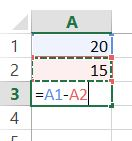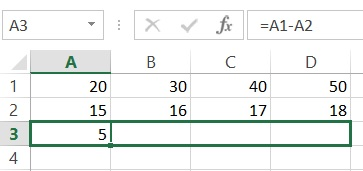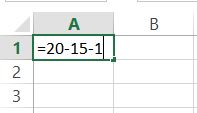#### How to subtract in Excel

In this Excel tutorial, learn how to subtract.

Excel lets you count complex things and perform simple math, like subtraction.

## Subtraction in Excel

To subtract in Excel, use “-” or “SUBTRACT” function. Here are the steps:

### Using the “-” operator:

This is the most common way to subtract in Excel.

To subtract two cells, enter the following formula in the cell where you want the result to appear:

`=A1-B1`

where A1 and B1 are the cells that contain the values you want to subtract.

### Using the “SUBTRACT” function

The SUBTRACT function is a more versatile way to subtract in Excel. It can be used to subtract any number of values, including cells, numbers, and text. The syntax for the SUBTRACT function is as follows:

`=SUBTRACT(value1, value2, ...)`

where value1 is the first value to subtract, value2 is the second value to subtract, and so on.

### Using the AutoSum button

The AutoSum button is a quick way to sum a range of cells. To use the AutoSum button, select the range of cells that you want to sum and then click on the AutoSum button in the toolbar.

Excel will automatically add a formula to the selected cells that sums the values in the range.

## How to calculate number difference in different cells?

If the numbers are in different cells, we first check their markings. In our case, we want to subtract the number in cell A2 from the number in cell A1. So go to the next cell – A3 – and on the formula bar, type: = A1-A2, then press Enter.The result of the subtraction appears in cell A3.

If we want to immediately calculate the difference of consecutive pairs of cells, click the square in the selection of cell A3 and drag it right:In the same way, you can subtract more numbers if you have more cells. Then we enter in the same way =A1-A2-A3 etc.

## How to calculate number difference in one cell?

In some cases, we may want to calculate the difference right away without having to enter numbers into many different cells. To do this, in the cell itself, we enter the subtract formula, e.g. = 20-15-1 and press Enter.The result will appear in the cell. Note that the formula still appears in the formula bar, where you can edit it as needed.